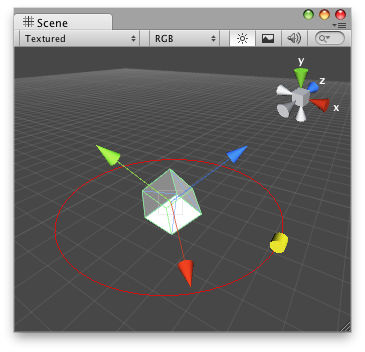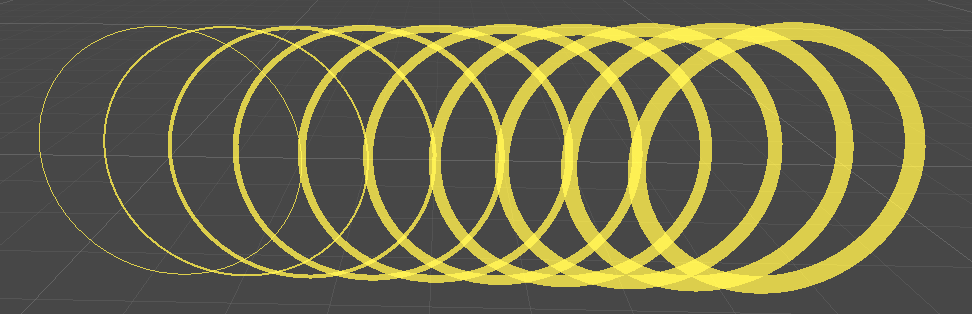Version: 2021.3

# Handles.DrawWireDisc

マニュアルに切り替える
public static void DrawWireDisc (Vector3 center, Vector3 normal, float radius, float thickness= 0.0f);

## パラメーター

 center The center of the disc in world space. normal The normal of the disc in world space. radius The radius of the disc in world space units. thickness Line thickness in UI points (zero thickness draws single-pixel line).

## 説明

Draws the outline of a flat disc in 3D space.

The Handles.color and Handles.matrix properties colorize and additionally transform the disc position. Unity ignores `DrawWireDisc` (that is, nothing happens) when the current GUI event type is not Repaint.Wire Disc in the Scene View.

```using UnityEngine;
using UnityEditor;// draw a red circle around the scene cube
[CustomEditor(typeof(CubeExample))]
public class CubeEditor : Editor
{
void OnSceneGUI()
{
CubeExample cubeExample = (CubeExample)target;        Handles.color = Color.red;
Handles.DrawWireDisc(cubeExample.transform.position, new Vector3(0, 1, 0), cubeExample.circleSize);
}
}
```

The cube:

```using UnityEngine;public class CubeExample : MonoBehaviour
{
public float circleSize = 3.0f;
}
```

You can use HandleUtility.GetHandleSize to calculate a suitable size for a manipulator handle.

Disc line `thickness` can be optionally set. Zero thickness draws a one-pixel line. Larger thickness values express line thickness in UI points. For example, a thickness of 1.0 could be two pixels wide on screen if the display zoom is 200% (see EditorGUIUtility.pixelsPerPoint).Discs of varying thickness.

```using UnityEngine;
using UnityEditor;public class ExampleScript : MonoBehaviour
{
}// Displays circles of various thickness in the scene view
[CustomEditor(typeof(ExampleScript))]
public class ExampleEditor : Editor
{
public void OnSceneGUI()
{
var t = target as ExampleScript;
var tr = t.transform;
var position = tr.position;
Handles.color = Color.yellow;
for (int i = 0; i < 10; ++i)
{
Handles.DrawWireDisc(position + Vector3.right * i, Vector3.forward, 2, i);
}
}
}
```